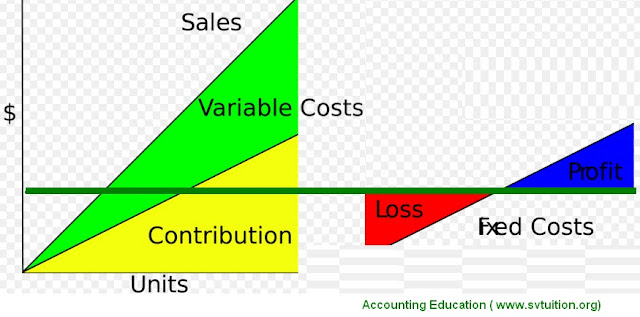In management accounting, we can use contribution margin for evaluate any product from financial point of view. It can be simply calculated by deducting variable cost from total sale revenue of any product.

1st Step : To Calculate Sale Price of Any Product

For example, cost of price of my management accounting eBook is Rs. 1000. I will not sell one management accounting ebook from this price.

2nd Step : To Calculate Variable Cost Any Product

a) For Selling this ebook, I use payment gateway. For example, if it deduct 10% commission on per sale. For example, I produced 1000 ebooks in Jan. 2017 and sold. It means, I had received earning Rs. 10,00,000. It means, I have paid commission to payment gatway Rs. 1,00,000.
So first variable cost is Rs. 1,00,000

b) For checking order, talking with customer and sending ebook and manage queries, I have appointed employees. For example, I am paid Rs. 1,00,000 to him.

c) Other Variable Cost for doing this specific work is Rs. 1,00,000

So, variable cost per unit is = Rs. 3,00,000 / 1,000 = Rs. 300 per unit

3rd Step : Calculate Contribution Margin

Contribution Margin = Sale price per unit - Variable Cost per unit

= RS. 1,000 - Rs. 300 = Rs. 700

If our contribution margin is more than our fixed cost, it is good sign because after deducting it, we can increase our scale of profit. Because whole one month, fixed cost will not increase. If fixed cost is very high than contribution, then, we will face loss. For this, either, we need to increase our price or leave same product.

We can also calculate Contribution margin % by using following formula.

Contribution margin % = Contribution margin per unit / sale price per unit X 100Comments on this Website
.

## \$type=three\$a=hide\$cm=hide\$s=hide\$show=/2019/06/10-steps-to-become-better-investor.html\$l=hide

Name

false
ltr
item
Accounting Education: How to Calculate Contribution Margin
How to Calculate Contribution Margin
https://1.bp.blogspot.com/-LkkOr0Mrbdc/WK7tQLG1vCI/AAAAAAAAVEE/PMGy3_tHte4Fr1atoVK_T79IhRkaAefPQCLcB/s640/effect%2Bof%2Bcontribution.TIF
https://1.bp.blogspot.com/-LkkOr0Mrbdc/WK7tQLG1vCI/AAAAAAAAVEE/PMGy3_tHte4Fr1atoVK_T79IhRkaAefPQCLcB/s72-c/effect%2Bof%2Bcontribution.TIF
Accounting Education
https://www.svtuition.org/2017/02/how-to-calculate-contribution-margin.html
https://www.svtuition.org/
http://www.svtuition.org/
http://www.svtuition.org/2017/02/how-to-calculate-contribution-margin.html
true
2410664366776677676
UTF-8